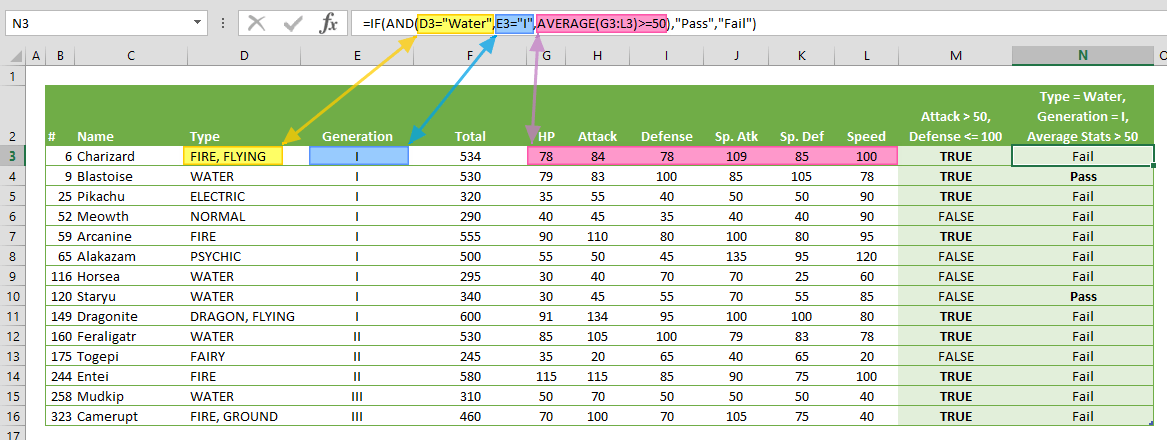Excel AND function is a logic comparison function that returns TRUE, if all specified conditions are met, and FALSE, if they're not. It In this guide, we’re going to show you how to use Excel AND function and also go over some tips and error handling methods.

# Supported versions

• All Excel versions

# Syntax

AND(logical1, [logical2], …)

# Arguments

 logical1 The first condition or logical test. logical2 Optional. Additional conditions or logic tests, up to a maximum of 255 conditions.

Examples

## Example 1

=AND(84>50,78<=100)
formula evaluates defined cases, and in this example returns TRUE, since all tests satisfy the conditions.## Example 2

=IF(AND("FIRE, FLYING"="Water","I"="I",AVERAGE({78,84,78,109,85,100})>=50),"Pass","Fail")
returns Fail because the first test ("FIRE, FLYING"="Water") returns FALSE. Like the AVERAGE function, other functions can be used in conjunction with any number of AND functions.# Tips

• Cheat sheet
 Calculation Returns TRUE AND TRUE TRUE TRUE AND FALSE FALSE FALSE AND FALSE FALSE
• Use the Excel AND function to extend the capabilities of other functions that use logical tests for parameters, like the IF function.
• Combine with other logical functions (OR, NOT, XOR) to generate even more complex scenarios.
• Comparison operators:
 Operator Description Criteria Sample Criteria Meaning = Equal to “=10000” Equal to 10000 <> Not equal to “<>10000” Not equal to 10000 > Greater than “>10000” Greater than 10000 < Less than “>10000” Less than 10000 >= Greater than or equal to “>=10000” Greater than or equal to 10000 <= Less than or equal to “<=10000” Less than or equal to 10000

# Issues

## #VALUE!

Excel AND function returns #VALUE! error if an argument doesn't provide a Boolean value.

## Empty cells

Empty cells are ignored by the AND function.